# How to build a Mortgage Calculator?

For the paper and pencil mathletes out there, the mortgage payment calculation looks like this:
M = P [ i(1 + i)^n ] / [ (1 + i)^n – 1]

Where the variables are as follows:

• M = monthly mortgage payment
• P = the principal amount
• i = your monthly interest rate. Your lender likely lists interest rates as an annual figure, so you’ll need to divide by 12, for each month of the year. So, if your rate is 5%, then the monthly rate will look like this: 0.05/12 = 0.004167.
• n = the number of payments over the life-span of the loan. If you take out a 30-year fixed rate mortgage, this means:- n = 30 years x 12 months per year, or 360 payments.

Pro Tip: Find more details about the calculations here

## The process

Now, let’s understand how you can replicate this formula to create a mortgage calculator using Outgrow.

For the starters, you’ll need the following inputs from the users:

Q1 – Loan Amount

Q2 – Annual Interest Rate

Q3 – Loan Duration in years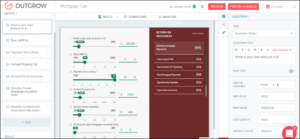### Monthly Payment:

Q1x(Q2/1200x(1+Q2/1200)^(Q3x12))/((1+Q2/1200)^(Q3x12)-1)

Note: Here we have divided Q2 by 1200 because Q2 is annual interest rate in percentage so if Q2 is monthly then we will divide it by only 100. Similarly, Q3 is payment terms in years, we have multiplied it by 12 to make it monthly.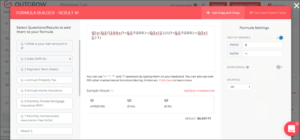In the formula builder –

• Add prefix and suffix as required. In this example, we have added ‘\$’ as a prefix.
• You can also add decimal places. Here we have added up to 2 decimal places.
• You can quickly validate the formula if the sample result displayed is correct.

### Total Interest Paid:

Q1x(Q2/1200x(1+Q2/1200)^(12xQ3))/((1+Q2/1200)^(12xQ3)-1)xQ3x12-Q1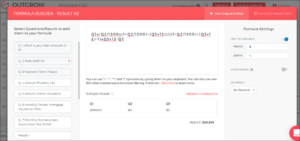### The total number of payments:

12*Q3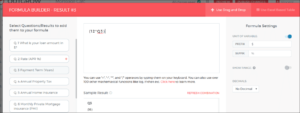### Total Mortgage Payments:

Q1x(Q2/1200x(1+Q2/1200)^(12xQ3))/((1+Q2/1200)^(Q3x12)-1)xQ3x12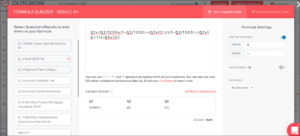We hope this makes it clear. Do you want to see one in action – check out this mortgage calculator below –